# Civil Engineering - UPSC Civil Service Exam Questions

26.

An equilateral triangular plate is immersed in water as shown in the above figure. The centre of pressure below the water surface is at a depth of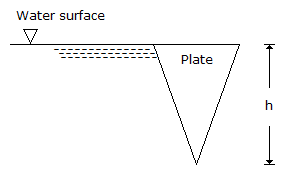A.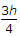B.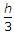C.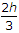D.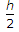Explanation:

No answer description available for this question. Let us discuss.

27.

Consider the following factors :
1. Air traffic control measures
2. Aircraft traffic composition
3. VFR/IFR operation
4. Runway configuration
Which of these factors affect the capacity of a runway ?

 A. 1, 2 and 3 B. 2 and 3 C. 1 and 4 D. 1, 2, 3 and 4

Explanation:

No answer description available for this question. Let us discuss.

28.

A city supply of 15000 cubic metres of water per day is treated with a chlorine dosage of 0.5 ppm. For this purpose, the requirement of 25% bleaching powder per day would be

 A. 300 kg B. 75 kg C. 30 kg D. 7.5 kg

Explanation:

No answer description available for this question. Let us discuss.

29.

The ratio between the stress produced in a bar by a sudden application of load (impact loading) as compared to the stress produced by the gradual application of same load is

 A. 1.5 B. 2 C. 2.5 D. 3

Explanation:

No answer description available for this question. Let us discuss.

30.

The shear stress distribution over a rectangular cross-section of a beam follows

 A. a straight line path B. a circular path C. a parabolic path D. an elliptical path